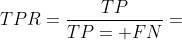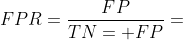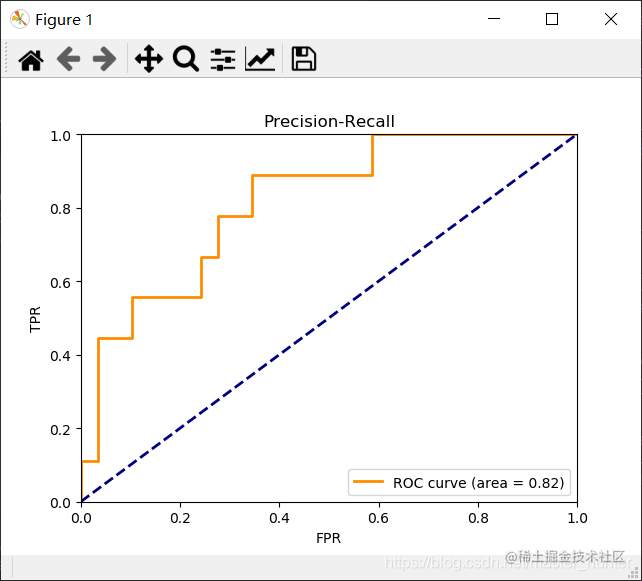# 一、ROC与AUC

## 1.ROC

ROC的全称是“受试者工作特征”曲线，与P-R曲线相似。与P-R曲线使用查准率、查全率为纵、横坐标不同，ROC曲线的纵轴是“真正例率”{简称TPR），横轴是“假正例率”（简称FPR）二者分别定义为：ROC曲线图以真正例率为Y轴，假正例率为X轴。

# 二、代码实现

from sklearn import svm, datasets from sklearn.model_selection import train_test_split from sklearn.metrics import roc_curve, auc from itertools import cycle from sklearn.preprocessing import label_binarize #标签二值化LabelBinarizer，可以把yes和no转化为0和1，或是把incident和normal转化为0和1。 import numpy as np from sklearn.multiclass import OneVsRestClassifier iris = datasets.load_iris()

X = iris.data

y = iris.target

# target是一个数组，存储了data中每条记录属于哪一类鸢尾植物，所以数组的长度是150,所有不同值只有三个

random_state = np.random.RandomState(0)

# 给定状态为0的随机数组

y = label_binarize(y, classes=[0, 1, 2]) n_classes = y.shape

n_samples, n_features = X.shape X = np.c_[X, random_state.randn(n_samples, 200 * n_features)]

# 添加合并生成特征测试数据集

X_train, X_test, y_train, y_test = train_test_split(X, y, test_size=0.25, random_state=0)

# 根据此模型训练简单数据分类器

classifier = OneVsRestClassifier(svm.SVC(kernel='linear', probability=True, random_state=random_state))#线性分类支持向量机 y_score = classifier.fit(X_train, y_train).decision_function(X_test)

# 用一个分类器对应一个类别， 每个分类器都把其他全部的类别作为相反类别看待。

fpr = dict() tpr = dict() roc_auc = dict() for i in range(n_classes): fpr[i], tpr[i], _ = roc_curve(y_test[:, i], y_score[:, i]) #计算ROC曲线面积 roc_auc[i] = auc(fpr[i], tpr[i])

fpr["micro"], tpr["micro"], _ = roc_curve(y_test.ravel(), y_score.ravel()) roc_auc["micro"] = auc(fpr["micro"], tpr["micro"]) import matplotlib.pyplot as plt

plt.figure() lw = 2 plt.plot(fpr, tpr, color='darkorange', lw=lw, label='ROC curve (area = %0.2f)' % roc_auc) plt.plot([0, 1], [0, 1], color='navy', lw=lw, linestyle='--') plt.xlabel('FPR') plt.ylabel('TPR') plt.ylim([0.0, 1.0]) plt.xlim([0.0, 1.0]) plt.legend(loc="lower right") plt.title("Precision-Recall") plt.show() 

## 效果